Performance Measures

# 11. Performance Measures

Much of the attention of real-world engineers is devoted to the exploration of alternative approaches to solving a problem at hand, each of which involves different tradeoffs between various cost and performance parameters. To approach these engineering tradeoffs systematically, it is important to have quantitative metrics of each.

Consider a digital system that performs some useful function: perhaps it plays digitally encoded music, shows a movie on a screen, or processes the payroll for a large corporation. Each of these applications has some set of performance requirements: the music player needs to generate output at some sampling rate in real time, and the payroll program must get the checks cut in time for payday. How these application-level requirements translate to choice of specific system structures and algorithms is the stuff of engineering.

Latency versus Throughput

## 11.1. Latency versus Throughput

To approach such questions, we abstract requirements on the performance of our digital components a bit. Consider a digital building block that computes some function, $P(x)$ for a given input value $x$. Given several implementations of devices computing $P(x)$, how do we compare their performance?

One obvious criterion is the answer to the simple question: given $x$, how long does it take to compute $P(x)$? In the case of combinational circuits, the answer is captured in the propagation delay specification $t_{PD}$. But we need to generalize the measure to arbitrary circuits containing clocked components and running in the discrete time that results from reticulating time into clock cycles. By this metric, the device that can produce the result $P(x)$ from an input $x$ in the shortest time is clearly the performance winner.

Depending on our application, however, this may not be the performance parameter that interests us. If we have a large number of independent inputs $x_1, x_2, ...$ that we need to process into results $P(x_1), P(x_2), ...$ we might care more about the rate at which a given device can compute $P(x)$ values from consecutive values of $x$.

Definition: The latency of a digital system is an upper bound on the interval between valid inputs to that system and its production of valid outputs.

The latency of a system is thus the time it takes to perform a single computation, from start to finish. The latency of a combinational device is simply its propagation delay $t_{PD}$; for clocked devices, the latency will be a multiple of the clock period.

Definition: The throughput of a digital system is the rate at which it computes outputs from new inputs.

The throughput of a system is the rate at which it accepts inputs, or, equivalently, the rate at which it produces outputs.

Since a combinational circuit can only perform a single computation at a time, it follows that the maximum throughput of a combinational circuit whose latency is $L$ is simply $1/L$, corresponding to the processing of a sequence of inputs by starting each new computation as soon as the prior computation has finished. The throughput measure becomes more interesting when we consider sequential circuits which may be performing portions of several independent computations simultaneously, a topic we visit shortly in Section 11.5.

## 11.2. Ripple-carry Binary Adder

Consider the problem of adding two $N$-bit binary numbers, for some constant width $N$. Although our focus here is on simple unsigned $N$-bit binary operands, any adder that works for $N$-bit unsigned binary operands will also correctly add signed $N$-bit operands represented in the two's complement form described in Section 3.2.1.2, a major attraction of the latter representation scheme.

An $N$-bit binary adder has at least $2 \cdot N$ bits of input and $N+1$ output bits, considering the two $N$-bit operands and a single output whose maximum value is twice the value of either operand. For reasonable input widths -- 16, 32, or 64-bit operands are common -- a combinational $N$-bit adder is a complex design problem. Fortunately, we can exploit symmetries in the structure of the problem to build viable $N$-bit adders by replicating a simple building block for each bit position of the sum.

### 11.2.1. Single-bit Adders

As a step toward adding $N$-bit binary numbers, consider the problem of adding a single bit of two $N$-bit binary numbers $A$ and $B$.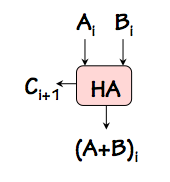We might devise a component such as the half adder shown on the right, whose inputs are the $i^{th}$ bits $A_i$ and $B_i$ of each operand, and whose output is the $i^{th}$ bit of the sum $A+B$. In defining such a module, we quickly realize that the sum $A_i + B_i$ requires two bits in its representation: the $i^{th}$ bit of the resulting sum, as well as a potential carry $C_{i+1}$ to the next higher-order bit position. This reflects the fact that the sum of the two input bits $A_i+B_i$ requires a two-bit representation, corresponding to the two outputs of the half-adder module.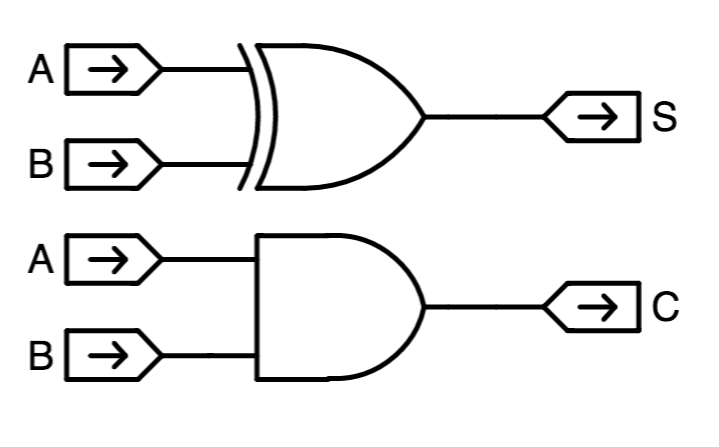We can implement the half adder module simply, using a 2-input XOR gate to compute the low-order bit $(A+B)_i$ of the sum, and a 2-input AND gate to compute the carry output $C_{i+1}$ as shown to the left.

Although a useful building block, the half-adder isn't quite the single-bit building block we need to replicate to make an $N$-bit adder. The half adder adds two single-bit inputs, producing a 2-bit sum: it produces a carry output for the next higher bit position, but lacks the additional input to accept a carry produced from an identical module in the next lower bit position. A bit of reflection convinces us that for a single-bit adder module to be cascadeable to add $N$-bit operands, carry bits must propagate between adjacent single-bit modules from low to high order bit positions.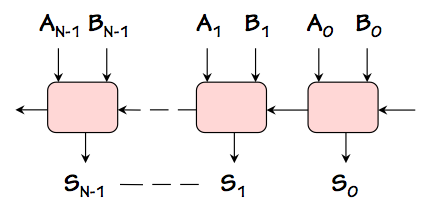We'd like a single-bit adder module that can be cascaded as shown to the right to make an $N$-bit adder; in addition to single operand bit inputs and sum bit output, the module needs a carry input from the lower-order bit to the right as well as a carry output to the higher-order bit to the left. Indeed, the requisite module must add three single-bit inputs ($A_i$, $B_i$, and $C_{in}$) to produce the two-bit sum ($C_{out}$, $S_i$).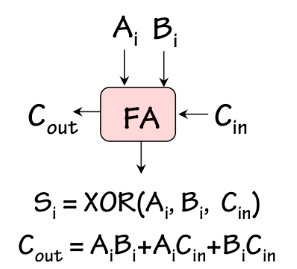The full adder, shown to the left, is a combinational device satisfying this specification. A ROM-based implementation of the full adder is shown in Section 7.8.3.2.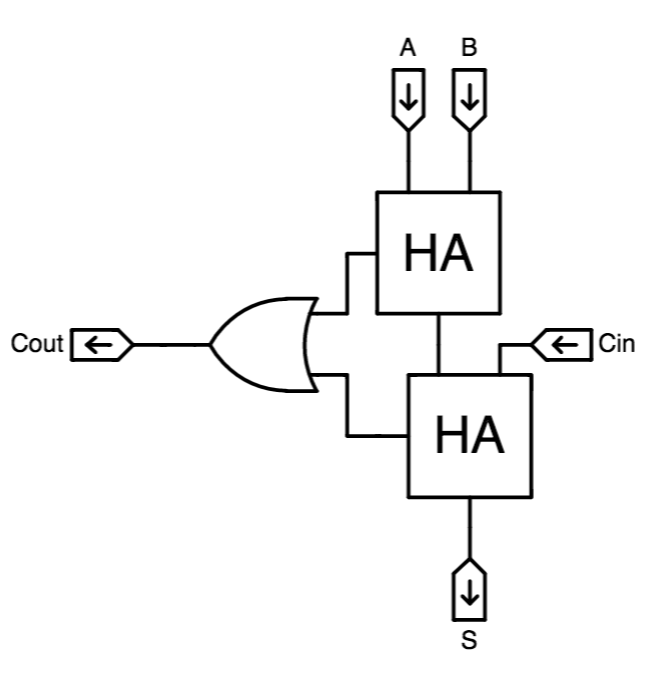It can also be implemented using two half adders -- hence its name -- as shown to the right. An $N$-bit adder can be made by cascading $N$ full adders as shown above, supplying the constant 0 as a carry into the low-order bit, and using the carry out of the high-order bit as the high-order bit $S_N$ of the sum. This design is called a ripple-carry adder, owing to the need to propagate ("ripple") the carry information from right to left along the cascaded carry in/out chain.

In fact, this carry propagation path is the performance-limiting feature of the ripple-carry adder. Suppose the propagation delay specification for our full adder is $t_{FA}$. Recall that this specification is an upper bound on the delay propagating any valid input to a valid output. Then the latency of our combinational $N$-bit ripple-carry adder -- its propagation delay -- is $N \cdot t_{FA}$, reflecting a worst-case propagation path from (say) $B_0$ to $S_{N-1}$. If we are interested in minimizing the latency of our $N$-bit adder -- which typically we are -- it is useful to specify the timing of our full adder in more detail. In particular, we might specify separate propagation delays for each input/output path, and ask how each effects the latency of the ripple-carry adder. If we enumerate all input/output paths through our $N$-bit adder, we find paths that include $N$ $C_{in} \rightarrow C_{out}$ delays, but at most one delay to a sum output bit. We conclude that, at least from this standpoint, the $C_{in} \rightarrow C_{out}$ propagation delay is the critical specification of our full adder implementation.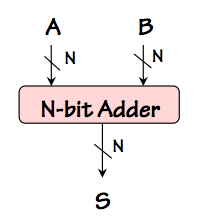We can abstract the function performed by our N-bit adder as a building block as diagrammed to the right: an $N$-bit adder module taking two $N$-bit binary numbers as inputs, and producing their $N$-bit sum as a result. Variations of this useful building block might offer an additional carry output (yielding an $N+1$-bit sum), and a carry input; for many purposes, however, we prefer simple adders that take and produce numbers of the same ($N$-bit) width.
Asymptotic cost/performance bounds

## 11.3. Asymptotic cost/performance bounds

It is often useful to characterize the behavior of cost or performance dimensions of a design as some parameter of the design, such as the size in bits of its inputs, becomes large. We might, for example, characterize the latency of a 2-input ripple-carry adder as growing in proportion to the number of bits being added. It is useful to abstract this growth rate, which reflects the architectural limits of the ripple-carry approach, from the actual propagation delay numbers of a particular adder design; the latter reflects particular technology choices as well as the architecture.

A common way to capture the abstracted growth rate of a cost or performance specification as some parameter (say $n$) becomes large uses one of several variations of the order of notation defined below:

Definition: We say $g(n) = \Theta(f(n))$, or "$g(n)$ is of order $f(n)$", if there exist constants $C_2 \geq C_1 \gt 0$ such that for all but finitely many integral $n \geq 0$, $$C_1 \cdot f(n) \leq g(n) \leq C_2 \cdot f(n)$$ We use the notation $g(n) = O(f(n))$ to specify that $f(n)$ satisfies the second of these inequalities for some $C_2$, but not necessarily the first.

The $\theta(...)$ notation specifies both upper and lower bounds on the growth it describes, while the weaker $O(...)$ constraint specifies only an upper bound. Thus the $O(...)$ notation is useful primarily for specifying upper bounds on costs (such as the time or space taken by an algorithm), and is commonly used in such contexts. The $\theta(...)$ is useful for specifying tight bounds on both costs (which we'd like to minimize) and performance parameters like throughput (which we'd like to maximize).

Note that the constant factors $C_1$ and $C_2$ in these definitions allow multiplicative constants to be ignored, while the finitely many exceptions clause effectively ignores additive constants in the growth characterization. One is left with a formula that captures the algorithmic form of the growth as a function of the parameter -- for example, whether it is linear, quadratic, polynomial, or exponential -- while ignoring complicating detail.

Some examples:

• $n^2+2\cdot n+3$ = $\theta(n^2)$, since $$n^2 \le n^2+2\cdot n+3 \le 2\cdot n^2$$ "almost always" (i.e., with finitely many exceptions);
• $log_2(n+7) = \theta(log(n)) = log_{10}(n+88)$, since the base of the logarithm and the added constants amount to constant factors;
• ${37589 ^ {34 \cdot 577} \over {\sqrt 17}} = \theta(1)$, as its value is constant (usually described as $\theta(1)$ or $O(1)$);
• $n^2 = O(n^3)$, but not $\theta(n^3)$, as the asymptotic growth of $n^3$ is an upper bound on that of $n^2$ but not a lower bound.
Asymptotic latency of N-bit Ripple Carry Adders

## 11.4. Asymptotic latency of N-bit Ripple Carry Adders

Returning to our N-bit ripple-carry adder, we quickly recognize that its asymptotic latency is $\theta (N)$ owing to the worst-case propagation path along the carry chain from low to high-order full adder modules. We have noted that the constant of proportionality is dictated by the propagation delay to the carry output of the full adder; minimization of this delay will improve the performance of the adder, but only by a constant factor. Improving the asymptotic performance, say to $\theta (log N)$, requires a more serious improvement to the architecture of the adder. We will explore such improvements shortly.

Before doing so, however, it is instructive to generalize our N-bit ripple carry adder to more than two operands. Given the $\theta(N)$ latency of our $N$-bit ripple-carry adder, it might seem sensible to add a large number $M$ of $N$-bit operands using a tree of $N$-bit adders, much like the tree of gates described in Section 7.7.2. If the latency of a two-operand $N$-bit full adder is $O(N)$, we could reason that a tree of such adders of depth $d$ would add $M = 2^d$ $N$-bit operands in $O(d \cdot N)$ time, yielding a latency of $O(N \cdot log M)$ bound for our $M$ operand by $N$ bit adder.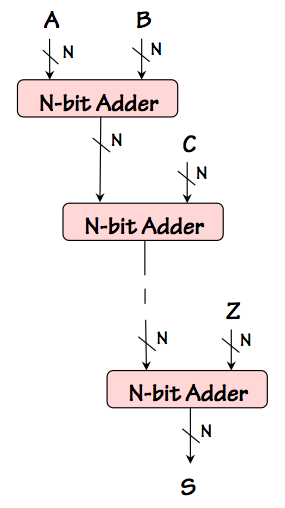This reasoning, however, uses only the worst-case latency characterization of our $N$-bit ripple carry adder and ignores the fact that some of its input-output paths are faster; a more detailed analysis yields a less pessimistic bound on the latency of our $NxM$ ripple-carry adder.

Consider a simple cascade of $N$ bit adders to compute $S = A+(B+(C ... +Z) ... )$ for some number of $N$-bit operands $A, ... Z$, as shown on the left. Again, given a cascade of $M$ $N$-bit adders each having a $O(N)$ latency, we might expect the latency of an $M$-operand adder constructed this way to exhibit $O(N \cdot M)$ latency. While $O(N \cdot M)$ is a valid upper bound, a closer look reveals that there is no actual input-output path through this circuit whose cumulative propagation delay grows as $O(N \cdot M)$. This suggests that a more detailed analysis might lead to a tighter bound on the latency of the circuit.NxM Ripple Carry Adder
To see this, we can expand the adder cascade into a two-dimensional array of full adders as shown to the right, each row adding a new operand to the accumulated sum coming from the row above. Note that signals propagate through this array in only two directions: the carry signals propagate from right to left, while the sum signals propagate from top to bottom. There is no case where a signal propagates to the right or upward. While there are many input-output paths to be considered, each is made up of segments propagating to the left or downward.

We conclude, from this observation, that the worst-case input-output propagation delay grows in proportion to the sum of the width and height of the array. Since the width grows in proportion to $N$ and the height in proportion to $M$, we conclude that the longest propagation path -- the critical path that bounds the latency -- grows as $O(N + M)$ rather than $O(N \cdot M)$. Since we can actually demonstrate a path whose cumulative propagation grows as $N+M$, this bound is tight; thus we are entitled to characterize the latency of this adder as $\theta(N+M)$, using $\theta$ rather than $O$ to indicate both upper and lower bounds on the latency.

Pipelined Circuits

## 11.5. Pipelined Circuits

Consider a combinational circuit to compute some function $P(X) = H(F(X), G(X))$ given the value of the input $X$.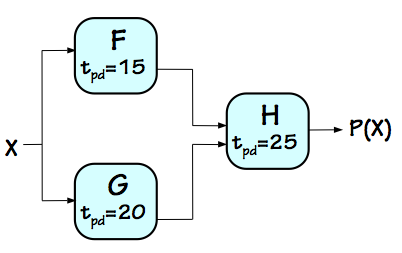Combinational P(X)
Assuming we have combinational modules that compute the component functions $F$, $G$, and $H$ with propagation delays of $15ns$, $20ns$, and $25ns$ respectively, the straightforward circuit on the left computes $P(X)$ with a latency of $45ns$. Note that for this discussion the time unit is arbitrary so long as it is used consistently. Since the combinational circuit can perform only a single $P(X)$ computation at a time, its throughput is simply the reciprocal of its latency $1/45ns$, or about $22.22$ MHz. This is simply the rate at which we can perform computations on our combinational device by presenting one input after another at the maximal frequency. If we have a large number $N$ of inputs $X_1, X_2, ... X_N$ for which we need to compute $P(X_1), P(X_2), ... P(X_N)$, we are likely to be more interested in throughput than in latency, as the total time for $N$ computations will be on the order of $L + N / T$ for throughput $T$ and latency $L$.

One observation we might make about our combinational circuit is that each of its components spends much of the $45ns$ propagation delay idle.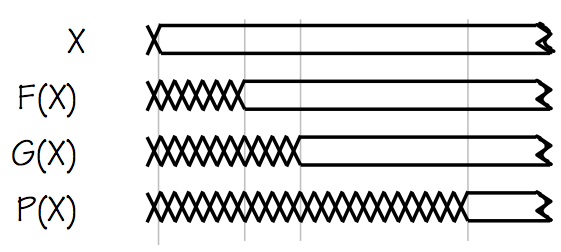P(X) Signal Timing
From the signal timing diagram on the left, we observe that the computation of $P(X)$ breaks down into two phases: during the first $20ns$ after a new value of $X$ is supplied, the $H$ component is waiting while $F(X)$ and $G(X)$ are busy computing their output values. During this phase, $H$ has invalid inputs and can do no useful computation. Once $F(X)$ and $G(X)$ are have become valid, a second phase is entered in which $H$ computes the output $P(X)$ while the $F$ and $G$ modules perform no useful computation other than maintaining valid inputs to $H$. This observation reveals that we are not fully utilizing the computational resources of the circuit's components: each component performs useful work, in the sense of computing new values from a newly-supplied input, during only one of these two phases of the computation.

We might be tempted to supply a new input $X_{i+1}$ during the second phase of the computation of $P(X_i)$, getting the jump on a new computation by the $F$ and $G$ modules while $H$ is completing the previous computation. However, a new input $X_{i+1}$ will contaminate the outputs $F(X_i)$ and $G(X_i)$ that are being used by $H$, which depends on valid inputs for the duration of the second phase. In order to guarantee a valid $P(X_i)$ value, we need to maintain valid, stable values at the inputs of $H$ during its entire propagation delay.

However, we can use relatively cheap registers to maintain stable values rather than combinational logic with stable inputs.Pipelined P(X)
If we divide our combinational circuit into two pipeline stages corresponding to the two phases of the computation, and put registers on all outputs of each stage as shown to the right, the result is a 2-stage pipelined circuit which performs the same computation as our combinational circuit. Following our single-clock synchronous discipline, all registers share a common periodic clock input. If we choose an appropriately long clock period, an input $X_i$ presented at the input near the start of clock cycle $i$ will cause the values $F(X_i)$ and $G(X_i)$ to be loaded into the registers at the outputs of the $F$ and $G$ modules at the start of clock cycle $i+1$, where they remain until the start of clock cycle $i+2$ when the value $P(X_i)$ is loaded into the register at the output of $H$.

If the timing parameters of the registers is negligible compared to the delay of other modules, we can do an approximate analysis assuming "ideal" (zero-delay) registers. Our first observation is that the minimum clock period that can be used for this pipelined circuit must be long enough to accommodate the longest combinational path in the circuit: in this case, the two paths through the $25ns$ $H$ module. Thus the fastest we can clock this circuit is a clock period of $25ns$, or a clock frequency of $40$ MHz. Since it takes two clock cycles to perform the computation of $P(X_i)$, the latency of our pipelined circuit is now $50ns$, somewhat longer than the $45ns$ latency of the original combinational circuit.

However, this pipelined circuit has the virtue that we can begin a new computation by supplying a new input on every clock cycle.Pipelined P(X) Timing
Since the computation of each $P(X_i)$ value takes two clock cycles, during each cycle each stage of the pipeline can be working on a different stage of an independent computation. The timing of consecutive computations is often summarized in a pipeline timing diagram like the one on the right, showing consecutive clock cycles on the horizontal axis and successive pipeline stages on the vertical axis. Note that at each active clock edge (vertical lines in the diagram) each $X_i$ computation moves to the next pipeline stage, progressing diagonally down through the diagram.

If we keep our pipeline full by supplying a new $X_i$ at each $i^{th}$ clock cycle (and reading the corresponding $P(X_i)$ at clock cycle $i+2$), the rate at which we are performing computations -- the throughput of our pipeline -- is just the clock frequency of $40$ MHz (the reciprocal of our $25ns$ clock period). Thus adding registers to convert our combinational circuit to a clocked pipelined version degraded the latency but improved the throughput, a tradeoff typical of pipelined circuits.

Any combinational circuit can be converted into a pipelined circuit performing the same computation by adding registers at carefully selected points. The addition of the registers actually lengthens input-output paths, so it actually increases latency; however, judicious selection of register locations can improve throughput significantly. The magic by which pipelining improves throughput involves (a) enabling a form of parallel computation by allowing the pipelined circuit to work on several independent computations simultaneously; (b) utilizing circuit components for a larger fraction of the time; and (c) breaking long combinational paths, allowing a high clock frequency.

Well-formed Pipelines

### 11.5.1. Well-formed Pipelines

Simply adding registers at arbitrary locations within a combinational circuit does not generally increase throughput; in fact, indiscriminant insertion of registers will likely change the computation performed by the circuit in undesirable ways.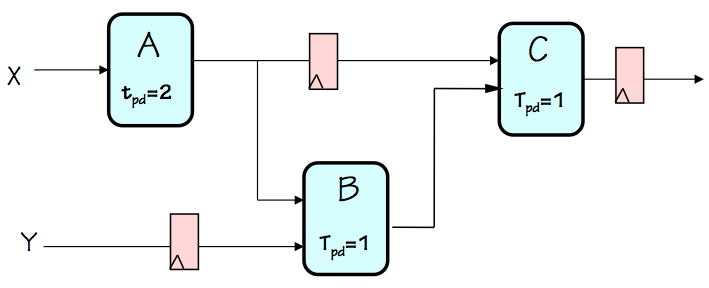Ill-formed pipeline
Consider, for example, the circuit on the left, a failed attempt at pipelining a combinational circuit made using combinational components $A$, $B$, and $C$. It was created from a working combinational circuit that computed, for each $X_i, Y_i$ input pair, an output value $C(A(X_i),B(Y_i, A(X_i)))$ by adding three registers. The added registers, however, delay the progression of input values by varying numbers of clock cycles as they propagate through the circuit. Imagine a sequence of input pairs $(X_1, Y_1), (X_2, Y_2), ...$ being applied to the $X$ and $Y$ input terminals on successive clock cycles, in an attempt to perform the computation on a succession of independent inputs. From the diagram, we see that the inputs to the $B$ module on a given clock cycle will be the values $A(X_i)$ and $Y_{i-1}$; the $B$ module will compute some confused value by mixing inputs from supposedly independent computations! We conclude that the circuit is not pipelined in the sense of our earlier, happier example; it does not perform the same computation as its orignal combinational version, inproving throughput by overlapping different phases of successive computations.

The problem illustrated by this ill-formed pipeline is that it fails to cleanly separate values associated with one set of inputs from those of another: values from successive computations interfere with each other as they progress through the circuit. Happily, a simple discipline allows us to avoid this problem: a necessary and sufficient condition for a well-formed pipeline is that it have an identical number of registers on each input-output path. By this standard, our failed example is not a well-formed pipeline.

We formalize this fact in the following definition:

Definition: A K-Stage Pipeline ("$K$-pipeline", for short) is an acyclic circuit having exactly $K$ registers on every path from an input to an output.

Note that by this definition, a combinational circuit is a $0$-pipeline.

It is useful to view a $K$-stage pipeline for $K>0$ as $K$ cascaded pipeline stages, each consisting of combinational logic with a single register on each output. This view leads to our convention that every pipelined circuit ($K$-pipeline for $K>0$) has one or more registers on every output. Such a convention is useful for a number of reasons; it allows, for example, a $k$-pipeline and a $j$-pipeline to be cascaded to create a $(k+j)$-pipeline.

For our synchronous pipelines (adhering to the single-clock synchronous discipline), the period $t_{CLK}$ of the shared clock must be sufficient to cover the longest combinational delay (plus register propagation and setup times). In this case,

• the latency of the $K$-pipeline is $K \cdot t_{CLK}$;
• the throughput of the $K$-pipeline is the frequency of the shared clock, or $1 / t_{CLK}$;
• and
• Inputs for the $i^{th}$ computation are presented during clock cycle $i$, and corresponding outputs are available during clock cycle $i+K$.
These rules characterize the externally-observable behavior of a $K$-stage pipeline: it takes a new input each clock cycle, and returns the corresponding output $K$ cycles later. This implies that the circuit maintains internally the state of $K$ simulaneous but independent computations: at every instant, it has $K$ latent outputs represented within its $K$ pipeline stages.
Pipeline Methodology

## 11.6. Pipeline Methodology

Given the constraint that a well-formed pipeline has the same number of registers on every input-output path, it is useful to develop a systematic method to convert an arbitrary combinational circuit to a well-formed pipeline offering improved throughput. While there are many possible approaches to this task, we sketch here an intuitive, informal technique that serves simple pipelining problems well.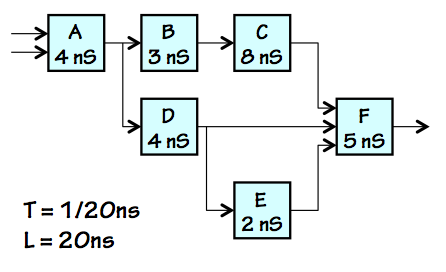Combinational Version
Our problem is to start with a combinational circuit, such as that shown on the left, and convert it to a $K$-pipeline that performs the same computation by inserting registers at appropriate points. The choice of "appropriate points" for the registers raises two separable issues: (a) ensuring that the result is indeed a well-formed $K$-pipeline for some value of $K$; and (b) ensuring that the pipelined circuit actually offers better throughput than the original combinational version. We deal with these two issues in the following sections.
Ensuring well-formedness

### 11.6.1. Ensuring well-formedness

Note that each component of the combinational example is marked with its propagation delay, and that the diagram is laid out so that all signal propagation is left-to-right. While the latter constraint is not essential to our technique, it makes the pipelining task easier and less error-prone.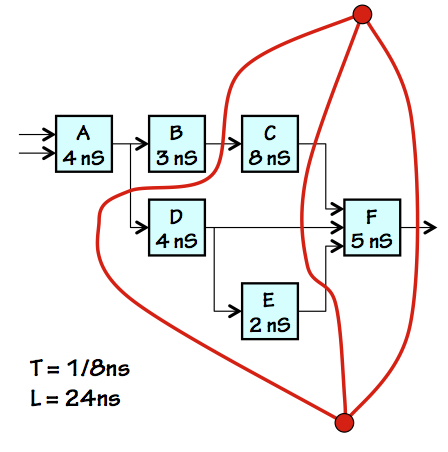3-Stage Pipelined Version
Our basic approach is to annotate our combinational circuit by drawing contours through it that identify boundaries between pipeline stages, as shown in red on the diagram to the right. Each red contour slices through a set of signal lines in the diagram, and thereby partitions the combinational circuit such that its inputs are all on one side of the contour and its outputs are on the other. It is important that the data on the signal lines all cross the contour in the same direction.

Each contour we draw identifies the output edge of a pipeline stage; thus, the first contour we establish is one that intersects all outputs of the combinational circuit. Subsequent contours are strategically chosen to break long combinational paths, improving throughput. We then make a pipelined version of our combinational circuit by inserting a register at every point where a signal line crosses a pipeline contour. Every input-output path thus crosses each contour exactly once; given $K$ contours, the result is necessarily a well-formed $K$-pipeline.

Notice that, with our choice of pipeline boundaries leading to the 3-stage pipeline shown, the longest combinational path (and consequently shortest clock period) is $8ns$. Given $3$ stages at an $8ns$ clock period, our pipeline has a latency of $3 \cdot 8ns = 24ns$ and a throughput of $1 / 8ns = 125 MHz$.

To summarize our technique for generating a $K$-stage pipeline from a combinational circuit:

1. Draw a first pipeline contour that intersects every output of the combinational circuit. This provides a template for implementation of a $1$-stage pipeline, usually an uninteresting implementation choice.
2. Draw additional contours determining boundaries between pipeline stages. Each contour must (a) partition the circuit by intersecting signal lines, and (b) intersect lines so that every signal crosses the contour in the same direction. Each additional contour increases the number of pipeline stages by one.
3. Implement the pipelined circuit by inserting a register at every point in the combinational circuit where a signal line crosses a pipeline contour.
4. Choose a minimal clock period $t_{CLK}$ (equivalently, a maximal clock frequency) sufficent to cover the longest combinational path within the circuit. Unless ideal registers are assumed, such paths must include the propagation delay of source registers as well as setup times of destination registers.
5. The result is a $K$-stage pipeline (where $K$ is the number of contours) whose throughput is $1 / t_{CLK}$ and whose latency is $K \cdot t_{CLK}$.
Optimizing Throughput

### 11.6.2. Optimizing Throughput

Not every well-formed pipeline offers a performance improvement over its combinational counterpart, or over alternative pipelines with fewer stages (and hence less hardware and performance cost). In general, it is worth adding a pipeline stage only when that stage reduces the critical (longest delay) combinational path, and consequently allows a faster clock and correspondingly higher throughput.To illustrate, consider the simple 3-component combinational circuit diagrammed to the left. Again, the latency (propagation delay) of each component is marked. We notice that the latency of the circuit, dictated by the cumulative propagation delay along its longest input-output path, is $4$, with a consquent throughput of $1/4$. It is instructive to look at this circuit, and propose how it might be pipelined to maximize its throughput. For this simple example, we will suffer the tedium of exploring reasonable possibilities exhaustively, assuming ideal (zero delay) registers.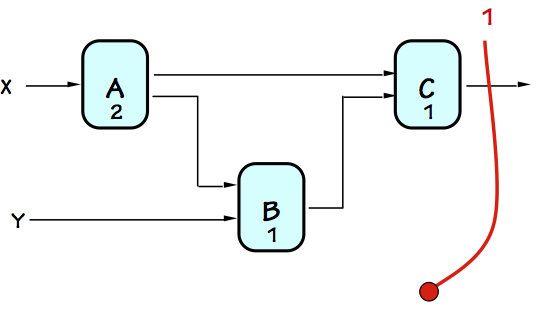Since our convention requires registers on each output of any pipelined circuit, the first pipeline stage boundary we draw intersects each output of the combinational circuit as shown to the right. The resulting circuit (after adding registers at intersections of our red contour with signal lines) is a well-formed 1-stage pipeline. Although this is a valid pipelined circuit, since registers are added only at its outputs, no combinational paths are shortened. Since the longest combinational path still encounters a propagation delay of $4$, $4$ is the shortest clock period that can be used with our 1-stage pipelined version, yielding a latency of $4 \cdot 1 = 4$ and a throughput of $1 / 4$ assuming ideal registers. Our single-stage pipelining gained no performance, and cost us some hardware (for the registers). If we assumed real (rather than ideal) registers, the added register propagation delays and setup times would slow the clock a bit further, actually lowering performance relative to the combinational circuit.The opportunity for real performance enhancement comes when we add a second pipeline stage, as indicated by the second contour in the diagram to the left. Here we have noticed that the $A$ component has twice the delay of the $B$ and $C$ components, and have chosen a pipeline stage boundary which isolates the slow $A$ from the faster components. Assuming ideal registers, the longest combinational delay in this $2$-stage pipeline is $2$, allowing a clock period as short as $2$ time units. This yields a latency of $2 \cdot 2 = 4$ and a throughput of $1/2$, doubling the rate at which the circuit can perform independent computations.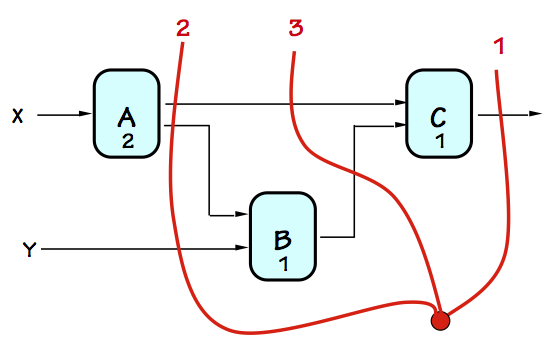Encouraged by the performance improvement gained by our $2$-stage pipeline, we might expect additional stages to further improve throughput. To the left we show a contour adding a third pipeline stage, defining a valid $3$-stage pipelined version of our original combinational circuit. However, we note that the longest combinational path within this circuit remains $2$, so the clock period remains $2$ and the throughput $1/2$ like that of the $2$-stage pipelined version. However, the third stage increases the latency to $2 \cdot 3 = 6$, degrading the performance from the cheaper $2-$stage version.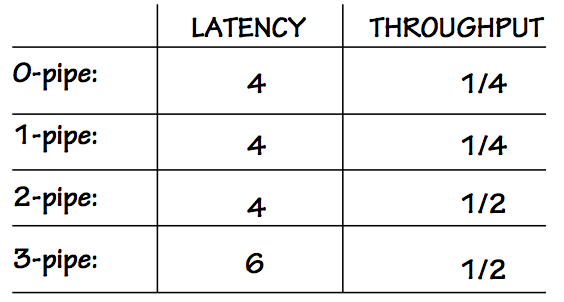The performance characteristics of the four versions of our circuit are summarized in the table to the left. It is worth observing that since the clock period (and hence the throughput) of any pipeline is limited by the longest combinational delay in the circuit, it is limited by the propagation delay of the slowest combinational component in that circuit. If the slowest components are isolated in separate pipeline stages and the clock period chosen to barely cover the propagation delays of these bottleneck components, there is no performance advantage to adding further pipeline stages.
Hierarchical Pipelines

## 11.7. Hierarchical Pipelines

The slowest-component bottleneck illustrated in the previous section can be mitigated by replacing slow combinational components with pipelined equivalents. Suppose, in the example of the previous section, it were possible to replace the combinational $A$ component whose latency of $2$ constrains the clock period with a $2$-staged pipelined $A'$ module capable of operating at a clock period of $1$.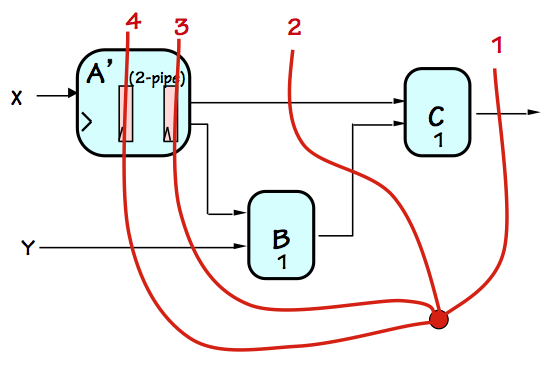The resulting circuit could operate as a $4$-stage pipeline, sustaining a clock period of $1$, a throughput of $1/1$ and a latency of $4 \cdot 1$ as shown on the right. Note that we have drawn two pipeline contours through the $2$-stage pipelined $A'$ module, reflecting the two registers it includes on each input-output path.

In general, it is straightforward to incorporate pipelined components into pipelined systems. Given a pipelined system whose performance is limited by the propagation of a specific combinational component, pipelining that bottleneck component is often the preferred solution.

Component Interleaving

### 11.7.1. Component Interleaving

It is not always practical to pipeline a bottleneck combinational component in a design, either for technical or practical reasons relating, for example, to the inaccessibility of the internals of a critical component. The bottleneck $A$ module of the prior example might be a black box library module whose supplier offers no pipelined equivalent nor grants engineering access to its implementation details. Such a constraint would seem to restrict us to a clock period of 2 and correspondingly low throughput of our system.

Fortunately, there are alternative strategies to get around this performance bottleneck. We begin by the simple observation that if one instance of some component $X$ offers throughput $T_X$, we can use $N$ instances of $X$ running in parallel to achieve an aggregate throughput of $N \cdot T_X$. We may need some surrounding logic to route inputs to and outputs from our farm of parallel $X$ modules, but clearly the throughput of any module can be scaled up by simple replication.

Armed with this insight, we might ask how to double the throughput of the bottleneck $A$ module in our example by using two $A$ modules rather than a single one. It would be convenient, for example, if we could use two $A$ modules to build a plug-in replacement for $A$ whose external behavior is precisely that of a 2-stage pipelined $A$ module. Such a replacement could simply be used as a component in the 4-stage pipelined approach shown above.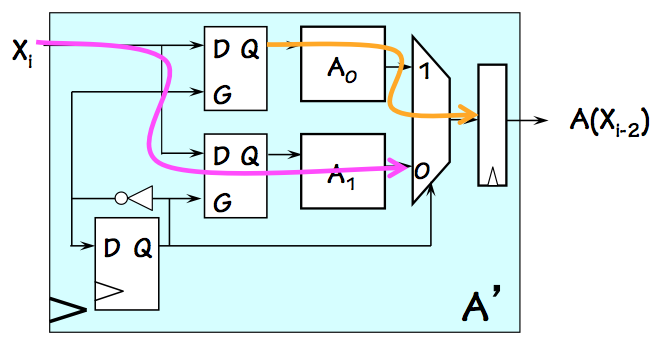2-way Interleaved Module
One approach to building pseudo-pipelined components involves interleaving the execution of replicated instances of that module, using circuitry like that shown at the right. This circuit uses two instances of the $A$ module, labeled $A_0$ and $A_1$, each with a propagation delay of $2$ to emulate a $2$-stage pipelined $A'$ module that can be clocked with period 1. Note that the flip flop in the lower left corner flips value on each active clock edge, distinguishing between even and odd cycles.

The two latches connected to the $X_i$ input latch input data on even and odd cycles, respectively, and hold the data as stable output for the nearly two clock cycles it takes their $A$ module to compute a valid result. During an odd clock cycle, $Q=1$ and the current $X_i$ input is routed through the lower latch and $A_1$, stopping at the output mux (purple arrow); meanwhile the $X_{i-1}$ input latched during the previous clock cycle remains in the upper latch, where it propagates through $A_0$ and the output mux (yellow arrow) to be loaded into the output register at the next active clock edge. The roles of the latches alternate every cycle, giving each $A$ component the required two cycles to complete its computation. Consistent with the specifications of a two-stage pipeline, however, the 2-way interleaved module takes a new input on every clock cycle and produces the corresponding output two cycles later.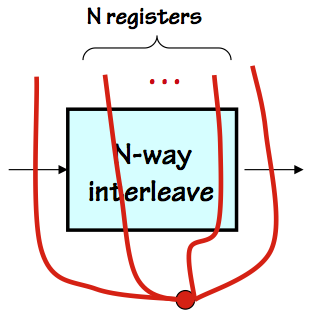N-way Interleaving
The interleaving technique can be generalized to emulate the behavior of an $N$-stage pipeline by replicating $N$ copies of a combinational module. An $N$-way interleaved module can be incorporated into a pipelined hierarchy by allowing $N$ pipeline contours to intersect the interleaved module, implying the presence of $N$ registers on each input-output path through that module. While that implication may not be literally valid (as our 2-stage interleaved module illustrates), its critical behavioral characteristic is preserved: an $N$-clock delay between presenting inputs and appearance of corresponding outputs.
Pipeline Issues

### 11.7.2. Pipeline Issues

Any combinational circuit can be partitioned into well-formed pipeline stages, with potential gains in throughput. Since the throughput of a pipelined circuit is constrained by its clock frequency, we generally maximize throughput by minimizing the longest combinational path within any pipeline stage. The clock period chosen for such a pipeline must be sufficient to cover this propagation delay plus the propagation delay and setup time of the registers used for the pipelining.

As we increase the number of pipeline stages to improve throughput, the cost and delay of the pipeline registers themselves may dominate other design parameters. Consider a complex combinational circuit constructed entirely from 2-input NAND gates having a $t_{PD}$ of 10ps. Pipelining this circuit for maximal throughput would place at least one pipeline stage boundary between every pair of connected gates, allowing the resulting circuit to be clocked with a period of 10ps plus the register $t_{PD}$ and setup times. If these were 35ps and 5ps, respectively, the minimum clock period would be 50ps, for a throughput of 20 GHz. This design would be very costly, owing to the number of flip flops required. Allowing for a 2-gate delay in each stage would cut the number of stages in half, yet allow a 60ps clock cycle for a marginally lower throughput at dramatically reduced cost.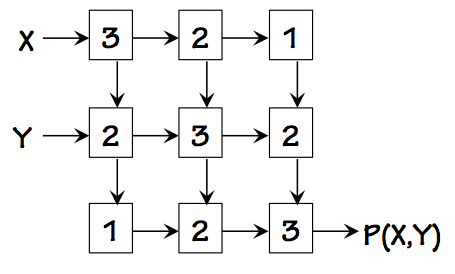Combinational P(X,Y)
Consider a 9-component combinational circuit to compute some function $P(X,Y)$ from $X$ and $Y$ inputs, shown in the diagram to the left. Each block is a (different) combinational component, and is marked with its propagation delay. Arrows on the interconnections indicate the direction of signal flow; the diagram is drawn so that signals flow generally down and to the right. If we were asked to pipeline this circuit for maximum throughput, we might begin by determining what the target throughput might be. Since the slowest components in the diagram have a propagation delay of 3, we recognize that pipeline boundaries within this circuit will not allow a clock period below 3, even using ideal (zero-delay) registers; so our goal becomes drawing pipeline boundaries such that the worst-case combinational path has a cumulative delay of 3.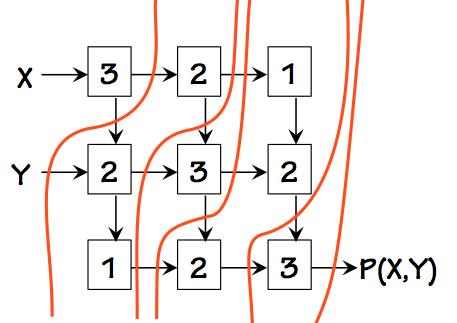One way to do so is shown to the right. Note that, per our convention, one pipeline boundary goes through each output of the circuit (of which there is only one in this case, marked $P(X,Y)$). Each of the slow components marked 3 is isolated in a pipeline stage, although there are several stages that have paths through two components whose total propagation delay is 3. A total of five stages is necessary to get the clock period down to 3. Note that our well-formed pipeline construction requires a register on the $Y$ input but none on $X$. It also places back-to-back registers, with no intervening logic, on several wires. While this may seem wasteful such patterns are commonly required to assure that every input-output path has the same number of registers.
Alternative Timing Disciplines

## 11.8. Alternative Timing Disciplines

As we combine sequential (clocked) components to make bigger sequential systems, we need an engineering discipline to control the timing of operations by each component, and to integrate their effects into a coherent system behavior. While our examples will focus on simple, widely used approaches, it is worth noting that there are reasonable alternatives whose usefulness and popularity vary among application arenas, engineering cohorts, and chapters of the history of digital systems engineering. In this section, we briefly sketch the universe of timing disciplines and several interesting variants on our simple synchronous approaches.
Synchrony vs. Localization

### 11.8.1. Synchrony vs. Localization

In our taxonomy of control disciplines, we distinguish between two separable engineering choices:
• synchronous versus asynchronous systems, the distinction being whether signal changes are synchronized with respect to a common clock (synchronous) or may happen at arbitrary times (asynchronous).
• globally versus locally (or self) timed systems, an architectural choice between centralized logic for controlling the timing of component operations and distributing the logic among the components themselves.
These two choices divide the universe of timing disciplines roughly into four quadrants, of which three have serious followings among the engineering community and are discussed in subsequent sections. The fourth, Asynchronous Globally-timed Systems, involves centralized generation and distribution of signals whose timing is critical to system integrity; it is generally frowned upon in modern engineering practice.
Synchronous Globally-timed Systems

### 11.8.2. Synchronous Globally-timed SystemsSGT System
The widely-used Synchronous Globally Timed engineering discipline depends upon a common clock signal to synchronize the timing of signal transitions throughout the system; it allows use of the single-clock synchronous timing scheme described in Section 8.3.3. Variants may partition the system into several clocking domains, each having its own shared clock signal.

Individual components may have control signals, whose changes are synchronized with the common clock. A simple example is the Load Enable input that dictates whether a register loads new contents at the next clock edge. The globally-timed aspect of this approach reflects a centralized source of these control signals, typically a FSM.

Synchronous Locally-timed Systems

### 11.8.3. Synchronous Locally-timed Systems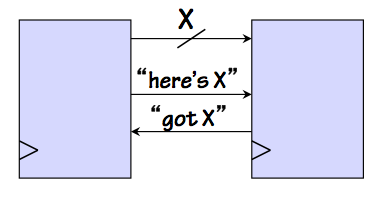Example SLT Protocol
Synchronous Locally-timed (or self-timed) systems retain the shared clock, and remain consistent with the single-clock discipline. However, locally-timed systems involve a component-level signaling discipline by which each component is told when to start an operation and reports when it has finished, as shown to the left.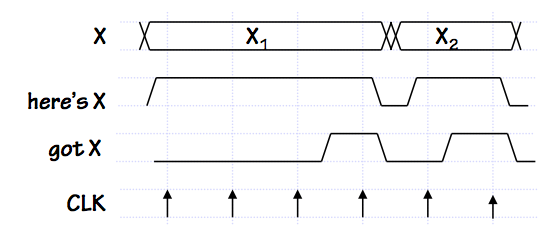SLT Signal Timing
In this illustration, communication of data $X$ between two interconnected modules is controlled by two signal lines: a "here's X" signal asserted by the source of the $X$ data to indicate the cycle on which it becomes valid, and a "got X" response from the recipient of $X$ to indicate that it has been safely loaded and no longer must be asserted by the source. A negative transition on "here's X" constitutes an acknowledgement that the "got X" has been noted, and the $X$ value is no longer asserted. In this example protocol, "got X" is asserted for a single cycle and automatically returns to zero in the following cycle. Because this is a synchronous protocol, each of these control signals must obey setup and hold time constraints with respect to the common clock.

Locally-timed systems generally involve additional logic that combines component timing signals to stimulate appropriate system-level timing. A glimpse of such logic appears in Section 11.8.4.1.

The elegant idea that underlies locally-timed systems is that the time taken for each operation is determined by the component that performs that operation, rather than by some externally specified plan. Each component will begin a new operation when modules supplying input data signal that the required inputs are ready, and will signal modules depending on its output when the computation has completed. Potential advantages of locally-timed system architectures include

• The timing of an operation, along with the implementation details of that operation, are enclosed in a single component. That component can be viewed externally as a "black box": knowledge of its internal details and timing are unnecessary for its effective use as a component. Thus, locally-timed component provides a more effective abstraction than one whose timing details must be part of its specification.
• Upgrading a component of a locally-timed system with an improved, faster version may improve the system performance with no additional changes. The new module will signal readiness of its output sooner, adjusting the timing of subsequent operations automatically.
• A locally-timed component can exploit a more sophisticated timing model than a conventional alternative. The model may be data dependent: for example, a self-timed multiplier might complete quickly when one of its operands is zero, allowing system timing to exploit this shortcut without any external provision in the system design.
For these and related reasons, self-timed protocols (both synchronous and asynchronous) have enjoyed a cult following among the engineering community, and are often utilized in situations where their abstraction and adaptivity advantages are critical. However, they come at some engineering cost: typically they replace timing decisions that are made during the system design with logic that makes these decisions dynamically during system operation, complicating component design and imposing certain hardware and performance costs.

Asynchronous Locally-timed Systems

### 11.8.4. Asynchronous Locally-timed Systems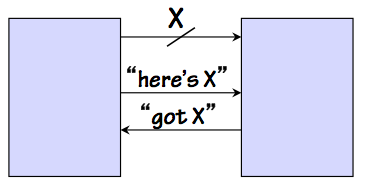Example ALT Protocol
Asynchronous locally-timed systems share the "have/got" control signals used to handshake sequences of data along a data path, but abandon dependence on the clock: the detailed timing of the transfer is dictated by the timing of the control signal edges themselves.

Typically the "have/got" signals follow a protocol similar to that described for the synchronous locally-timed systems, where each of the control signals makes both a positive and a negative transition for every data transfer in a sequence. This convention requires two transitions on each control signal line per transfer, imposing an engineering challenge since control signals change at twice the frequency of the data values. An elegant alternative is to use a single transition signalling protocol: the flow of data $X$ is controlled by a "have X"/"got X" control signal pair where any transition on either control line -- positive or negative -- signals the corresponding "have X" or "got X" event.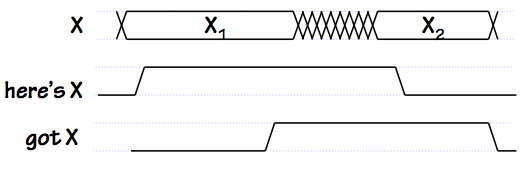Single-transition Signalling
This scheme halves the frequency of control signal changes with respect to the SLT protocol shown above: note, in the timing diagram to the right, a single transition on each control signal for each new value of $X$ passed.

Locally-timed Example

#### 11.8.4.1. Locally-timed Example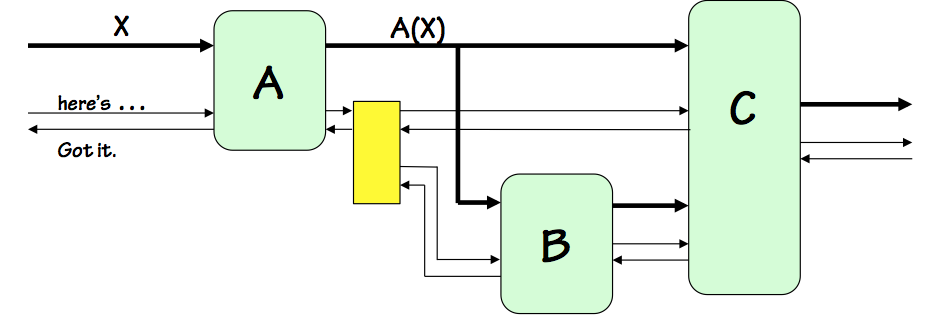Self-timed Example
As a brief glimpse of the organization of a locally-timed system, consider the Asynchronous Locally-Timed system shown to the right. We assume an asynchronous (clockless) signaling scheme using "have" and "got" signals that make two transitions to handshake each data transfer, as described in Section 11.8.3.

The system itself behaves as a locally-timed component following the same handshake protocol; its input $X$ has "here's X" and "got X" control lines to control the flow of data in, and its single output is likewise associated with control signals in either direction to handshake data to its destination. Within the system itself, every data path has a pair of associated control signals allowing the data source to signal readiness of the data, the destination to signal readiness to accept the data, and so on.

Since the system components are locally-timed and follow the same protocol, each internal data path is associated with a pair of "here's" and "got" control signals between the source and destination of the data. A complication arises from the output of the $A$ componenent, which is routed to two destinations, $B$ and $C$. The pair of control signals associated with $A$'s output must be routed to control signal terminals of both the $B$ and $C$ modules, each a destination whose timing must be coordinated with $A$'s output timing. We show in the diagram a yellow box, representing a library fan-out circuit module associated with our timing discipline for routing a locally-timed data path to multiple destinations.Fan-out Module
Plausible circuitry for this module is shown to the right. The "have" signal for the source, asserted after the data output from $A$ has been made valid, can simply be routed to the destinations $B$ and $C$, announcing that their respective input data are valid. However, we require that $A$ maintain valid output data until both of these destinations have signaled that they no longer need it: a logical AND of the acknowledgements by $B$ and $C$ that they have received the data. The protocol requires the "got" signals to persist until the "here's" signal is dropped, assuring that both input "got" signals will be present simultaneously to generate the "got" output of the fan-out module.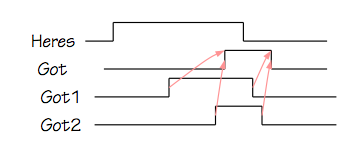Since the negative transition of the "got" signals indicated readiness for new data, we need to combine the "got" signals from $B$ and $C$ in such a way that $A$ sees a positive transition at the later of the two positive transitions, and a negative transition at the later of the negative transitions on the "got" signals from $B$ and $C$ as shown in the timing diagram to the left.

A computation of $C(A(X), B(A(X)))$ by our example locally-timed system would typically proceed as follows:

1. Initially the system is quiescent, and signals its readiness to accept a new input by a logical 0 on the "got X" control signal from $A$ to the component supplying $X$ data.
2. When new $X$ data becomes available, its source (connected to the $X$ data and control lines) drives valid input data on the $X$ lines, and signals its readiness to $A$ via the "have X" control signal.
3. The $A$ component recognises that it has valid data, and begins the computation of $A(X)$.
4. At some point after seeing the "here's X" signal, $A$ will no longer require valid input data and signal that via the "got X" line back to its source. This may happen early or late in the computation of $A(X)$, depending on the design of the $A$ module (which may, for example, load the $X$ value into an internal register to allow a faster "got X" response).
5. After the source of the $X$ data sees the "got X" signal, it will return "here's X" to 0 and stop driving the $X$ data lines.
6. Once the $A$ component has completed its computation, it drives the $A(X)$ result on its output and signals its validity to the $B$ and $C$ modules via the corresponding "here's" control signal.
7. Seeing the signal that its $A(X)$ input data is valid, the $B$ module begins its computation. Once again, $B$ may respond with a "got A(X)" signal at any point during its operation.
8. When $B$ has computed $B(A(X))$, it drives its output line and signals readiness to the $C$ module.
9. The $C$ module begins its computation after it has been signaled that its inputs from both $A$ and $B$ are ready. Again, it may respond with the appropriate "got" control signals at any point during its operation.
10. When both $B$ and $C$ signal they no longer need their $A(X)$ input, the fan-out logic signals "got A(X)" to $A$, which restores the "has A(X)" signal to 0 and stops driving the $A(X)$ data lines.
11. On completion of $C$'s computation, it drives its output data lines and signals "here's C(A(X),B(A(X)))" to external components taking this value as input.
Note that the order of these events is not necessarily fixed; modules can vary the timing of their "got" control signals to input sources as noted above. This flexibility allows, for example, individual components to be internally pipelined, so that a new $C(A(X),B(A(X)))$ computation might be started in $A$ while the previous computation is still being finished by the $B$ and $C$ components.
Chapter Summary

## 11.9. Chapter Summary

This chapter introduces two related but separate measures of the performance of a system: Latency, the end-to-end time taken for each individual operation, and Throughput, the rate at which operations are performed. These metrics can be used to characterize potential usefulness of a wide range of systems, including combinational and sequential digital information processing circuitry.

Latency and throughput of parameterized families of circuits, such as $N$-bit adders, may be characterized in asymptotic terms as parameters grow large using the $O(...)$ and $\theta(...)$ notations. The latency of an $M$-word by $N$-bit ripple-carry adder, for example, grows as $\theta(N+M)$.

Pipelining is introduced as a way of improving system throughput at some cost in latency. A $k$-pipeline is a circuit having:

• $k$ distinct pipeline stages, separated by registers on outputs of each stage;
• $k$ registers on every input-output path;
• potential to begin a new operation on each clock cycle, producing corresponding outputs $k$ cycles later;
• Latency of $k \cdot t_{CLK}$ and throughput of $1 / t_{CLK}$, where $t_{CLK}$ is the clock period.

Combinational circuits may be viewed as $0$-pipelines, a degenerate case whose latency is $t_{PD}$ and whose throughput is $1 / t_{PD}$.